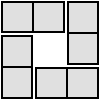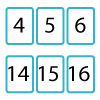#### You may also like### 4 Dom

Use these four dominoes to make a square that has the same number of dots on each side.### Twenty Divided Into Six

Katie had a pack of 20 cards numbered from 1 to 20. She arranged the cards into 6 unequal piles where each pile added to the same total. What was the total and how could this be done?### Mystery Matrix

Can you fill in this table square? The numbers 2 -12 were used to generate it with just one number used twice.

# Satisfying Four Statements

## Satisfying Four Statements

Ash, Si, Sami and Mani are playing a game.
Each of them writes down a statement that describes a set of numbers.

Ash writes "Multiples of five".
Si writes "Triangular numbers".
Sami writes "Even, but not multiples of four".
Mani writes "Multiples of three but not multiples of nine".

Can you find some two-digit numbers that belong in two of the sets?
Can you find some two-digit numbers that belong in three sets?
What is the smallest number that belongs in all four sets?

How could you describe the pattern of the numbers that satisfy both Ash's and Sami's statements?
How about the numbers that satisfy both Ash's and Mani's statements?

Can you describe patterns for other pairs of statements?

You might now like to play the game Statement Snap and then have a go at Charlie's Delightful Machine.

### Why do this problem?

This problem offers a starting point to explore properties of numbers, and provides a good introduction to the ideas developed in Factors and Multiples Puzzle and Charlie's Delightful Machine.

In conjunction with the other tasks in this group, this task also offers the chance to focus on any of the five key ingredients that characterise successful mathematicians

### Possible approach

You could begin the lesson by dividing the board into two columns, one headed with a tick and the other headed with a cross.
Ask students to suggest numbers, and write each suggestion in the appropriate column according to a rule of your own choice. Possible rules could be prime numbers, odd numbers, multiples of 4...
Once a few students think they know what your rule might be, invite them to make guesses that will help the rest of the class guess the rule too.

"Some friends played a game like this with two-digit numbers, and they used these four rules:

• Multiples of five
• Triangular numbers
• Even, but not multiples of four
• Multiples of three but not multiples of nine

Choose any two of the rules, and see if you can find some two-digit numbers that satisfy both of them."

Give students a little bit of time to find some examples, and then extend the problem...
"Now choose one of the other rules. Do any of your numbers satisfy that one too?"

Again, give students some thinking time. Then set the final challenge:
"Can you find a two-digit number that satisfies all four of the statements?"

Again, give students some time to work on this, then finish by bringing the class together to discuss how they know it's not possible to satisfy all four rules using two-digit numbers.

You could follow this up by asking students to consider how they would describe the numbers that satisfy pairs of statements:

"How could you describe the pattern of the numbers that satisfy both Alison's and Sam's statements?
How about the numbers that satisfy both Alison's and Matt's statements?

Can you describe patterns for other pairs of statements?"

### Key questions

Which rules have lots of numbers that satisfy them?
Which rules don't have very many numbers that satisfy them?
Where is it best to start if you're looking for numbers that satisfy more than one rule?

### Possible extension

Invite students to relax the two-digit number restriction - can they satisfy all four rules now?